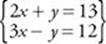﻿ ﻿THE SUBSTITUTION METHOD - Equations and Inequalities - ALGEBRA - SAT SUBJECT TEST MATH LEVEL 1

## ALGEBRA## CHAPTER 6Equations and Inequalities### THE SUBSTITUTION METHOD

If in a system of equations either variable has a coefficient of 1 or –1, solving the system by the substitution method may be just as easy or even easier than solving it by the addition method. Look at the system of equations you solved in Example 27:. By subtracting 2x from each side of the first equation, you can rewrite that equation as y = 13 – 2x. Now in the second equation you can replace y by 13 – 2x :

3x – (13 – 2x) = 12

This is now a simple equation in one variable that you can solve using the six-step method.Then substitute 5 for x :

y = 13 – 2(5) = 13 – 10 = 3

The solution is x = 5 and y = 3.

﻿